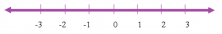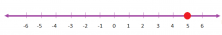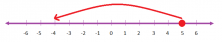Smartick is an online platform for children to master math in only 15 minutes a day

Mar17

In today’s blog post, we are going to look at integers: what they are and how to add and subtract them.

Let’s start by understanding what integers really are:

Integers are numbers that aren’t a fraction or a decimal. These numbers include natural numbers (1, 2, 3…), negative numbers (-1, -2, -3…) and zero (0).

Integers can be represented on the number line. On the right side of the zero, are the natural numbers and on the left side, we can find the negative numbers.#### Adding and Subtracting Negative Numbers

In order to learn how to add or subtract integers, we are going to use the number line.

1. We need to find the first number on the number line.
2. If we are adding, we count however many values the second number is towards the right.
3. If we are subtracting, we count however many values the second number is towards the left.

For example: 5 –9

So, we need to locate the first number on the line.If we are adding, we count however many values the second number is to the right. If we are subtracting, we count however many values the second number is to the left.

In this problem, we are subtracting, which means we need to count towards the left. The second number is 9, so we count 9 values to the left from 5.So, 5 – 9 = – 4

#### Trick:

I’m going to show you guys a small trick. I hope it helps you to add and subtract more easily.

• If the two numbers have the same sign, we add the two numbers without paying attention to the sign, and after adding, we’ll stick the sign that the numbers had back on the final answer. For example: – 2 – 5

Both of the numbers have the same sign, so we add them: 2 + 5 = 7. Now, we just add on the sign that the two numbers had to begin with, which is the negative sign (-). The final answer, then, is -7.

• If the two numbers have a different sign, we just subtract them: the bigger number minus the smaller number. After, we add on whichever sign the big number had onto the answer. For example: 3 – 7

Both of the numbers have different signs, so we subtract the smaller one from the bigger one: 7 – 3 = 4. Now, we just add on the sign the bigger number had: -7 (negative). Our final answer is -4.